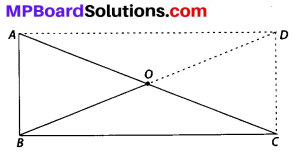## MP Board Class 8th Maths Solutions Chapter 3 Understanding Quadrilaterals Ex 3.4

Question 1.
State whether True or False.
(a) All rectangles are squares.
(b) All rhombuses are parallelograms.
(c) All squares are rhombuses and also rectangles.
(d) All squares are not parallelograms.
(e) All kites are rhombuses.
(f) All rhombuses are kites.
(g) All parallelograms are trapeziums.
(h) All squares are trapeziums.
Solution:
(a) No, because in a square all sides are equal, but it is not true in case of rectangle.
(b) Yes, because both diagonals bisect each other and opposite sides are equal.
(c) Yes, because in square all sides are equal.
(d) No, all squares are parallelograms. Because all squares satisfies the conditions of a parallelogram.
(e) No, because only two pairs of consecutive sides are equal in kite whereas in rhombus all sides are of equal length.
(f) Yes, all rhombuses are kites.
(g) Yes, because in trapezium, there is only one pair of parallel opposite sides and in parallelograms, two pairs of opposite sides are parallel.
(h) Yes, because in trapezium, there is only one pair of parallel opposite sides and in square, two pairs of opposite sides are parallel.Question 2.
Identify all the quadrilaterals that have
(a) four sides of equal length
(b) four right angles
Solution:
(a) The quadrilaterals, those have four sides of equal length are square and rhombus.
(b) The quadrilaterals, those have four right angles, are square and rectangle.

Question 3.
Explain how a square is
(ii) a parallelogram
(iii) a rhombus
(iv) a rectangle
Solution:
(i) A square is four sided, so it is a quadrilateral.
(ii) Since a square has opposite sides parallel and diagonals bisect each other, so it is a parallelogram.
(iii) Since square is a parallelogram with all 4 sides equal, so it is a rhombus.
(iv) Since square is a parallelogram with each angle a right angle, so it is a rectangle.Question 4.
(i) bisect each other
(ii) are perpendicular bisectors of each other
(iii) are equal
Solution:
(i) The quadrilaterals in which diagonals bisect each other are rhombus, rectangle, square and parallelogram.
(ii) The quadrilaterals in which diagonals are perpendicular bisectors of each other are rhombus and square.
(iii) The quadrilaterals in which diagonals are equal are square and rectangle.Question 5.
Explain why a rectangle is a convex quadrilateral.
Solution:
When we draw the diagonals joining the end points of a rectangle it lies in its interior. So, it is a convex quadrilateral.

Question 6.
ABC is a right-angled triangle and O is the mid point of the side opposite to the right angle. Explain why O is equidistant from A, B and C. (The dotted lines are drawn additionally to help you).Solution:
Since ∆ABC is right angled at B. So ∠D = 90°, $$\overline{A D} \| \overline{B C}$$ and $$\overline{A B} \| \overline{C D}$$
⇒ ABCD is a rectangle where AB = CD and AD = BC
AC and BD are the diagonals which bisects each other.
Thus AO = OC and BO = OD, also BO = OC which shows that O is equidistant from A, B and C.